# Two melons

There are 2 melons in the box. Together they weigh 11kg. The weight of green is 3 kg less than three times the weight of yellow. What is the weight of a green melon?

x =  7.5 kg

### Step-by-step explanation:

x+y = 11
x = 3•y-3

x+y = 11
x-3y = -3

x = 15/2 = 7.5
y = 7/2 = 3.5

Our linear equations calculator calculates it.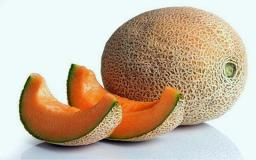Did you find an error or inaccuracy? Feel free to write us. Thank you!Tips to related online calculators
Do you have a system of equations and looking for calculator system of linear equations?

## Related math problems and questions:

• Children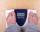Margaret and Zdena weigh the same and Petra 3 kg more. Together weigh 156 kg. How much do they weigh?
• HomeworkMother and Kate together weigh 24.5 kg more than the father. Father weighs 7.6 kg more than mother. Father weight 44.8 kg more than Kate. Determine weights of father mother and Kate.
• Barrel 4Barrel of water weighs 63 kg. After off 75% water, the weight of the barrel with water is 21 kg. How many kg weigh empty barrel and how many kgs water in it?
• Water wessel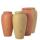The vessel containing water has a weight 19 kg. After pouring half the water its weigth 11 kg. What is the weight of the empty vessel?
• Weigh in totalI put 3/5 kg of grapes into a box which is 1/4kg in weight. How many kilograms do the grapes and the box weigh in total?
• Watering can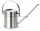A watering can full of water weighs 11 kg, the weight of water is 10 kg greater than the weight of an empty watering can, how much is the weight of the watering can?
• The devils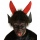The devils weighed in hell with Dorota. They found that Dorota and the two devils weigh 250 kg together, and Dorota and the four devils weigh 426 kg. All the devils weigh the same. How Much Does Dorota Weigh?
• Bent scaleMonica weighed 52 kg. Sara 54 kg. Together they weighed 111 kg. They noticed that the weight on the scale was bent. How much did they really weigh?
• Trio weight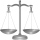Adelka, Barunka, and Cecilka are weight in pairs. Adelka with Barunka weighs 98 kg, Barunka with Cecilka 90 kg and Adelka with Cecilka 92 kg. How much does each of them weigh?
• Empty canisterThe canister is filled with oil weight 17 kg. If filled only half weight 9 kg. What weight does my empty canister have?
• Bags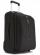The first bag has a weight of 18 kg, the second bag is a one-third lighter than the first and the third bag has three times less the weight of all three bags together. Determine their weights.
• Father and sonFather and son weigh together 108kg. The father weighs 2.6 times more than his son. How much does weight my father and son count?
• Six hensSix hens weigh 3 kg more than three geese and 7 kg less than five geese. How much does a hen weigh, and how many geese?
• Container with water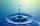The weight of a container with water is 2.48 kg. If the cast 75% water, the container with water has a weight of 0.98 kg. Determine the weight of the empty container. How much water was originally in the container?
• The water barrel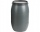The water barrel weighs 122 kg. If we pour 75% of the water out of it, it will weigh 35 kg. What is the weight of the barrel?
• BucketThe bucket half filled with water weighs 5.55 kg, the full bucket weighs 9.85 kg. How much does the bucket weigh?
• Can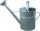Watering can full of water weighs 10 kg. Half-full can weighs 5.5 kg. How much weigh can?